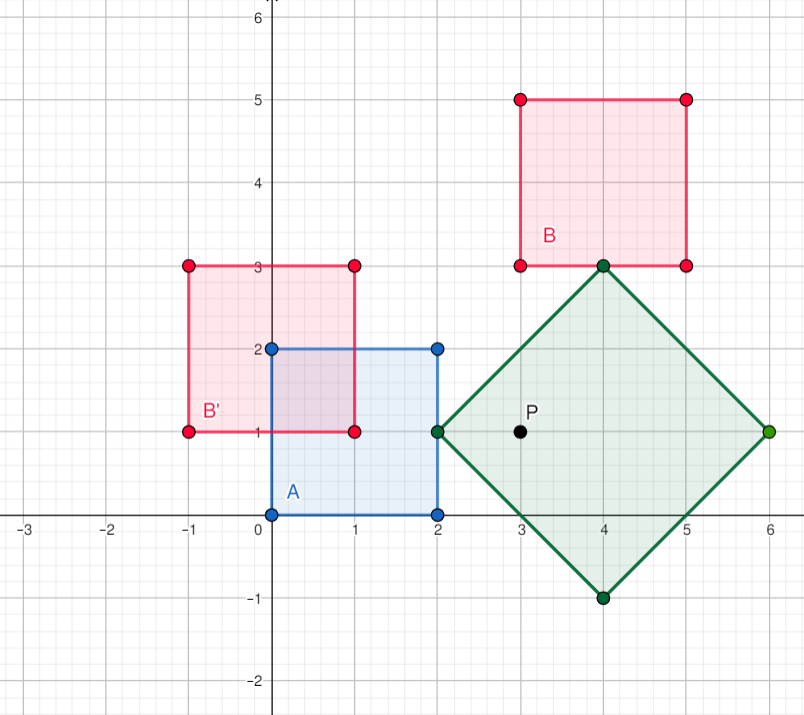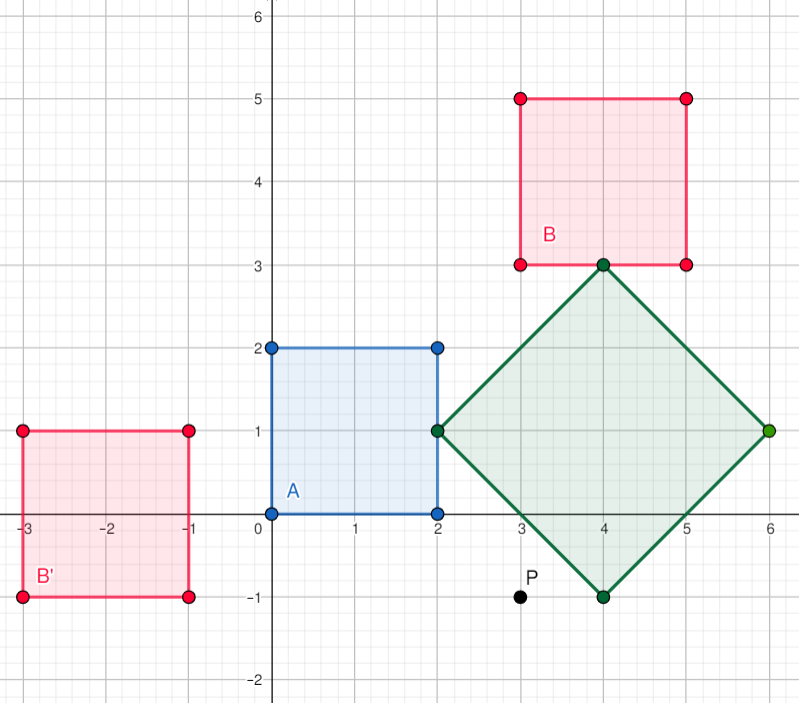# Rotation Dilemma

Limits 2.5s, 512 MB

Akash has two favorite convex polygons $A$ and $B$. But one of his new year resolution for 2020 is to have only one favorite polygon (not necessarily convex). So, he is in a dilemma and has given you the task to somehow merge these two polygons. Having received such a task, the first thing that came to your mind is to choose a point $P$ and rotate the polygon $B$, $D$ degrees counter-clockwise with respect to $P$. After the rotation, you are hoping that the new polygon $B'$ and $A$ will become one connected region, and this connected region will be Akash's new favorite polygon. Formally speaking, you are hoping that $A$ and $B'$ will have a non-empty intersection.

Of course, choosing any random $P$ will not get you this result. Still, there are many valid choices for $P$, in fact, all such choices form a bounded measurable shape (locus as a geometer would call it). So before choosing a $P$ and rotating the polygon, you decide to find the area of this shape/locus.

## Input

In the first line number of test cases $T$ ($0 < T \leq 5$) is given. Each test case starts with a line containing $D$ ($0 < D < 360$). Next, two polygons $A$ and $B$ will be described. For each polygon, the first line contains the number of points $n$ ($3 \leq n \leq 10^5$), next $n$ lines will contain coordinates $(x, y)$ ($|x|, |y| \le 10^7$) of the points. All input numbers will be integers. The points will be in counter-clockwise order. Note that the polygons are not necessarily strictly convex i.e. internal angles can be $180$ degrees but not more.

## Output

For each test case, output the area of the locus of P in a single line. Your answer will be considered correct if the relative or absolute difference is less than $10^{-6}$. That is, if your answer is $P$ and jury's answer is $Q$, your answer will be considered correct if $\frac{|P-Q|}{max(1, Q)} < 10^{-6}$.

## Sample

InputOutput
1
90
4
0 0
2 0
2 2
0 2
4
3 3
5 3
5 5
3 5

8.00000000


In the pictures below, the blue square is polygon A, red squares are B and B'. The green shape is the locus of P. note that when P is inside it, B' and A have a non-empty intersection. But when P is outside the green area, B' and A do not intersect.